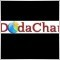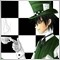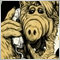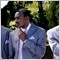# Calculating the difference in Pips62

Hello,

I need to calculate the difference in pips of two values.
e.g.

// some price
double var1 = 1. 3574 ;

// current price
double cprice = Close;

How to calculate the difference of pips in these variables, considering the decimal places used by different brokers.2760

`double DiffPips = MathAbs(NormalizeDouble(var1-cprice,Digits)/Point);`62

On UWC, it displays corrects e.g. 12 or 15.

But on Alpari account, it displays 120 or 150 on same values i.e. 10 time more.

Something is missing.

Thanks23253

int point_compat = 1;
if(Digits == 3 || Digits == 5) point_compat = 10;

double DiffPips = MathAbs((NormalizeDouble(((price1 - price2)/MarketInfo(Symbol(),MODE_POINT)),MarketInfo(Symbol(),MODE_DIGITS)))/point_compat);20207

gkdoda:
On UWC, it displays corrects e.g. 12 or 15.
But on Alpari account, it displays 120 or 150 on same values i.e. 10 time more.
On 5 digit brokers a point is 1/10 pip. EAs must adjust TP, SL, AND slippage from pips to price or points.
```//++++ These are adjusted for 5 digit brokers.
int     pips2points;    // slippage  3 pips    3=points    30=points
double  pips2dbl;       // Stoploss 15 pips    0.0015      0.00150
int     Digits.pips;    // DoubleToStr(dbl/pips2dbl, Digits.pips)
int     init(){
if (Digits == 5 || Digits == 3){    // Adjust for five (5) digit brokers.
pips2dbl    = Point*10; pips2points = 10;   Digits.pips = 1;
} else {    pips2dbl    = Point;    pips2points =  1;   Digits.pips = 0; }
// OrderSend(... Slippage.Pips * pips2points, Bid - StopLossPips * pips2dbl
}
string  DeltaToPips(double d){
if (d > 0)  string sign = "+";  else    sign = "";
double pips = d / pips2dbl;
string dPip = sign + DoubleToStr(pips, 0);  if(Digits.pips==0) return(dPip);
string dFrc = sign + DoubleToStr(pips, Digits.pips);
if (dPip+".0" == dFrc)      return(dPip);           return(dFrc);          }
string  PriceToStr(double p){
string pFrc = DoubleToStr(p, Digits);       if(Digits.pips==0) return(pFrc);
string pPip = DoubleToStr(p, Digits-1);
if (pPip+"0" == pFrc)       return(pPip);           return(pFrc);          }
```20207

calofe:
double DiffPips = MathAbs((NormalizeDouble(((price1 - price2)/MarketInfo(Symbol(),MODE_POINT)),MarketInfo(Symbol(),MODE_DIGITS)))/point_compat);

Why use unnecessary complicated function calls?

MarketInfo(Symbol(),MODE_POINT) == Point

MarketInfo(Symbol(),MODE_DIGITS) == Digits147

Hi Guys!

I want help with executing a buy when current tick price - previous tick price have a price gap of let's say 10pips to the upside. Meaning current tick price is greater than previous by 10pips

Enter a sell when current tick price - previous tick price have a price gap of let's say 10pips to the upside. Meaning previous tick price is greater than current by 10pips.

Price gap is what I want to use to enter and exit trades.

I have been cracking my head with this and I'm stuck. Please assist with code